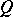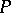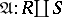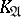# Associative calculus

Jump to: navigation, search

A name given to calculi (cf. Calculus) of a very definite type, especially suitable for specifying finitely-presented associative systems (semi-groups, cf. Semi-group). The term "associative calculus" was introduced by A.A. Markov, who also developed the theory of associative calculi .

Any associative calculusis defined by specifying some alphabetand a finite listof relations in— pairs of words (cf. Word) in this alphabet. The words constituting a relation are often called its parts — left and right. A-permissible operation on words inis any substitution of one part of any relation belonging to, where it occurs imbedded in a word in(cf. Imbedded word), by the other part of the same relation. An associative calculusis the performance of a sequence of-permissible operations, starting from any wordin. One says that all wordsobtained in this way (including the initial worditself) are equivalent toin the associative calculus(in symbolic notation,). The relationfor any associative calculusis reflexive, symmetric and transitive. In addition, it follows fromandthat. This makes it possible, in a natural manner, to assign to each associative calculusa finitely-presented associative system, whose elements are equivalence classes of words inand whose multiplication operation is induced by the operation of concatenation of words in. The associative systemthus constructed will have a unit (the element represented by the empty word); the elements ofrepresented by the letters of the alphabetwill constitute a system of generating elements for, while the list of relationsrepresents a complete system of relations between these generating elements ofin the sense that the elements ofrepresented by two wordsandare identical inif and only ifandare equivalent in. Thus, the recognition of the identity of elements inis reduced to the recognition of equivalence of words in. Hence the importance of the study of the solvability of the algorithmic problem of recognition of equivalent words in an arbitrary associative calculus. This problem was first formulated by A. Thue : Construct, for an arbitrary associative calculus, an algorithm which, for any pair of words in the alphabet of this associative calculus, determines, in a finite number of steps, whether or not the words constituting the pair are equivalent in. In the algebraic interpretation, this problem is the identity problem for the associative system. Thue succeeded in solving the problem in a few special cases only. In the modern interpretation of the problem (1930's and later) the algorithm of this problem is sought in some exact meaning of the word, such as a partial recursive function, a Turing machine or a normal algorithm. If the problem is thus modernized, the problem of finding a specific associative calculus for which the desired algorithm does not exist becomes meaningful. Markov  and E.L. Post , working independently of each other, constructed unsolvable associative calculi, i.e. associative calculi with an unsolvable algorithmic problem of recognition of word equivalence. These results yield a negative solution of Thue's problem in its modern form. However, if one accepts the Church thesis or any other equivalent assumption regarding the adequacy of recursive functions for rendering the concept of an algorithm more precise, one comes to the inevitable conclusion that Thue's problem in its original form as well has a negative solution for some concrete associative calculi.

The original examples constructed by Markov and Post were extremely complex. Simpler unsolvable associative calculi were subsequently presented. They include, for example, an associative calculus with seven very simple relations , and one with only three such relations . Thue's problem has been almost fully solved for the case of an associative calculus with one relation .

In a natural way one defines an isomorphism of one associative calculus into another and onto another . Of special interest from the algebraic point of view is the study of those properties of associative calculi that are invariant under isomorphisms; they are the properties of abstract associative systems. Markov , who based himself on his own studies on the recognition of word equivalence in an associative calculus, obtained a very general result, which yields a negative solution to practically all algorithmic problems concerning the basic classifications of associative calculi studied at that time. He showed, in particular, that ifis an isomorphism-invariant property of an associative calculus, if there is a unique associative calculus with the propertyand if there exists also an associative calculus not included in any associative calculus with the property, then the algorithmic problem of recognition of an associative calculus with the propertyamong other associative calculi is unsolvable for any alphabet with more than three letters. It immediately follows that algorithmic problems of recognition of uniqueness of associative calculi, finiteness of an associative calculus, the semi-group character of an associative calculus, inclusion in group-associative calculi, and isomorphism of pairs of associative calculi, are unsolvable for any alphabet with more than three letters. It was subsequently proved  that the set of pairs of isomorphic associative calculi is recursively enumerable; the method used in this connection also makes it possible to establish the recursive enumerability of the number of invariant properties of associative calculi.